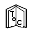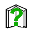## X3D Example Archives: VRML 2 Sourcebook, Chapter 19 Normals ShadingDirectory Viewpoint Slideshow
 Normal (perpendicular) vectors defined for individual points or polygons can change the shading of geometry. When shaded, the faces on a shape are obvious. Creating a smooth shape may require a large number of small faces. An alternative is to instead use smooth shading to create the illusion of a smooth shape, but with a smaller number of faces. The Normal node lists normal vectors to use for parts of a shape which can change shading. These are used as the value of the normal field, and normal index values select which normals to use (similar to color index values). Ordinarily the creaseAngle field controls faceted or smooth shading. Normals override the normal effect of the creaseAngle value. The normalPerVertex field selects normal per face/grid square or normal per coordinate. The NormalInterpolator node converts time values to normal values for animation effects. These X3D scenes are adapted directly from the original VRML 2.0 Sourcebook chapter examples. Also available: Introduction to VRML97 SIGGRAPH98 course notes.
9 X3D Models                 19 X3D Model Viewpoint SnapshotsFigure 19 21 Normal Square.x3dFigure19_21NormalSquare.x3d Normal square, solid=false means visible on both sidesFigure19_21NormalSquare.x3d (default X3D view from 0 0 10)Figure 19 22 Normal Square Shaded.x3dFigure19_22NormalSquareShaded.x3d Square with artificial normals, thus shaded. Careful, solid=true means visible only on one side!Figure19_22NormalSquareShaded.x3d (default X3D view from 0 0 10)Figure 19 23 Half Column.x3dFigure19_23HalfColumn.x3d Half column. Careful, only one side!Figure19_23HalfColumn.x3d (default X3D view from 0 0 10)Figure 19 24a Half Column Crease Angle.x3dFigure19_24aHalfColumnCreaseAngle.x3d Half column. Careful, only one side!Figure19_24aHalfColumnCreaseAngle.x3d (default X3D view from 0 0 10)Figure 19 24b Two Half Columns Crease Angle Seam.x3dFigure19_24bTwoHalfColumnsCreaseAngleSeam.x3d Two half columns - note visible seams where each side meetsFigure19_24bTwoHalfColumnsCreaseAngleSeam.x3d (default X3D view)Figure 19 25a Half Column Normals.x3dFigure19_25aHalfColumnNormals.x3d Half column. Careful, only one side! Normals are applied to vertical edges, but seam improvement not visible until halves put together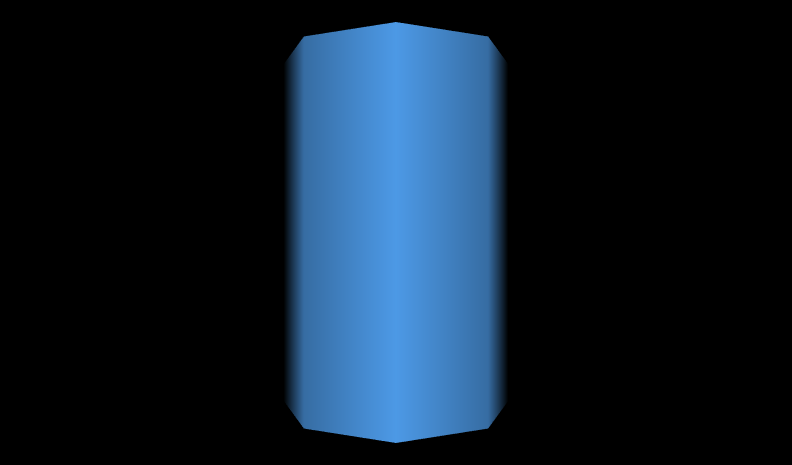Figure19_25aHalfColumnNormals.x3d (default X3D view from 0 0 10)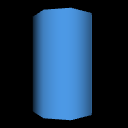Figure 19 25b Two Half Columns Normals Seamless.x3dFigure19_25bTwoHalfColumnsNormalsSeamless.x3d Two half columns - no more visible seams where each side meets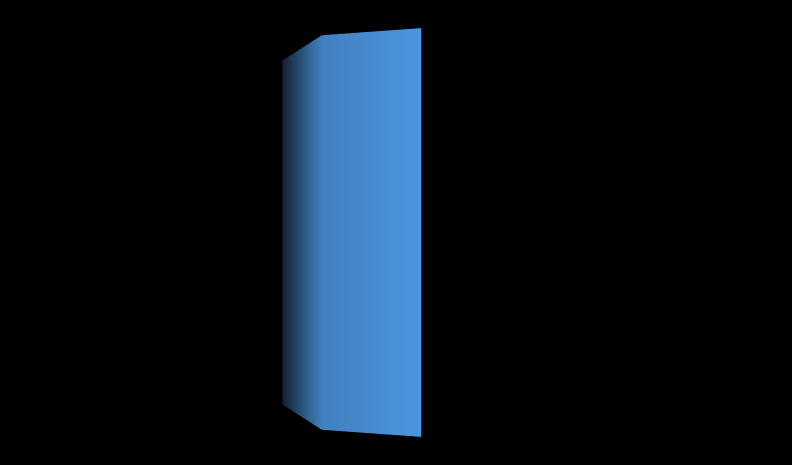Figure19_25bTwoHalfColumnsNormalsSeamless.x3d (default X3D view)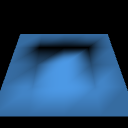Figure 19 26 Normal Shaded Elevation Grid.x3dFigure19_26NormalShadedElevationGrid.x3d Shaded (but flat) ElevationGridFigure19_26NormalShadedElevationGrid.x3d (default X3D view)Figure 19 27 Square Face Animating Normals.x3dFigure19_27SquareFaceAnimatingNormals.x3d Animating normals on right vertices of a quadrilateralFigure19_27SquareFaceAnimatingNormals.x3d Other side - note difference in animated shadingFigure19_27SquareFaceAnimatingNormals.x3d (default X3D view)

This viewpoint slideshow is online at